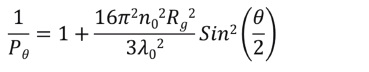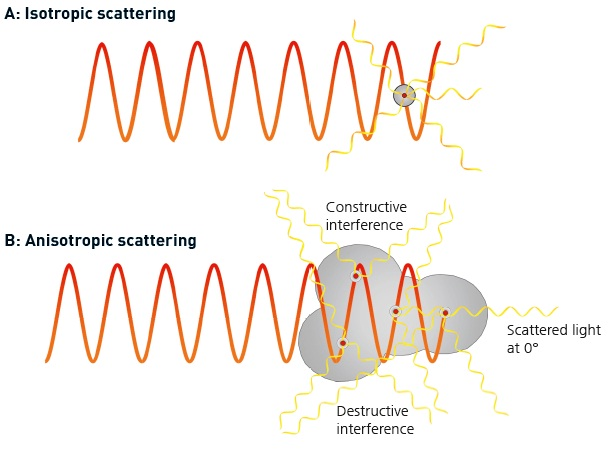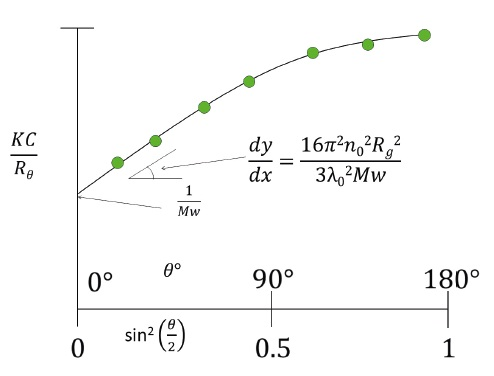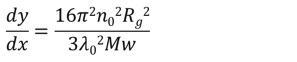# Angular Dependence and Light Scattering

Pθ, or form factor, is associated with molecular size and the angle at which light is scattered by the molecule, and is defined in the following equation:Where:

• n0 is the refractive index of the solvent,
• Rg is the molecule’s radius of gyration,
• λ0 is the laser wavelength in a vacuum,
• θ is the measurement angle.

According to the equation, 1/ Pθ relies on a number of factors associated with both the sample and the measurement, including the laser wavelength in a vacuum (λ0), the refractive index of the solvent (n0), the radius of gyration of the molecule (Rg) and the measurement angle (θ).

Hence the amount of light scattered by larger molecules will also rely on the measurement angle. This phenomenon is termed as ‘angular dependence.’

## Effect of Angular Dependence

For larger molecules, the scattered photons will no longer scatter independently, but rather will constructively or destructively interfere with one another, as depicted in Figure 1. If the size of the molecule is small compared to the incident laser beam, it behaves as a point scatterer, as illustrated in Figure 1A. In this case, the intensity of the scattered light from the molecule is even in all directions. These molecules are known as ‘isotropic scatterers.’Figure 1. A. An isotropic scatterer is small relative to the wavelength of the light and scatters light evenly in all directions. B. An anisotropic scatterer has significant size compared with the wavelength of the incident light and scatters light in different directions with different intensities.

As the molecule’s size increases with respect to the laser, the size and structure of the molecule come into play. Different points within the molecule scatter individual photons from the laser with varying phases, which allows the photons to interfere with one another. Hence, the resulting measured intensity will rely on the observation position. These molecules are called ‘anisotropic scatterers.’

If all of the interference is destructive, then the intensity will always appear to be lower than that for an isotropic scatterer. If this intensity value is used for the molecular weight calculation with the Rayleigh equation, the resulting value would be an underestimated value.

According to the Rayleigh equation, this effect can be eliminated if it is possible measure the scattering light intensity at a θ of 0°, at which the sin2(θ/2) would be ‘0,’ while the 1/Pθ would be ‘1.’

This means that at 0°, the scattering light intensity would be unchanged by the interference, thus enabling the relationship between intensity and molecular weight to be established in the same way that it is for the smaller molecules, though in these cases the scattered light is measured at a 90° angle.

However, it is not possible to isolate the purely scattered light at 0°, because at this angle the intensity of the illuminating laser is more powerful than the scattered light. Hence, it is not possible to make measurements at 0°, necessitating the requirement for an alternative technique.

At a diameter below 1/20th of the laser wavelength, the scattering of the light from the molecules will be isotropic, and above this level will be anisotropic. The typical laser wavelength in most instruments is in the range of 633-670 nm. Hence the limit of isotropic scattering is in the range of 15.8-16.8 nm radius. This nominal value of roughly 15 nm radius for the start of quantifiable angular dependence will also be the lower limit at which the molecular size (Rg) can still be reliably measured.

## Guinier Plot

The different types of light scattering instruments are engineered to address the angular dependence problem in different ways. To tackle the angular dependence problem, it is necessary to decide the value of the scattering light intensity at 0° by using the data from other angles. A Guinier Plot is an ideal way to calculate the value at 0°. A Guinier plot is a plot of KC/Rθ as a function of angle (sin2(θ/2)), as shown in Figure 2.Figure 2. A Guinier plot shows KC/Rθ as a function of angle (sin2(θ/2)).

Measurements of light scattering intensity are performed at the desired angle. The KC/Rθ values are calculated with the knowledge of the sample’s concentration and other factors and are plotted as shown in Figure 2 as a function of sin2(θ/2). The intercept of the line is 1/Mw, from which the molecular weight can be determined, and Rg can be calculated from the initial slope of the line as defined in the following equation:Nevertheless, the slope will be small and partially obscured by noise at small molecular sizes. Hence, a reliable Rg value can only be obtained if the molecule has an appreciable size compared to the laser light wavelength to yield a slope that is large enough.

## Conclusion

The nominal threshold value of Rg for a 633 nm laser is roughly 15 nm. Nonetheless, the absolute limit for Rg measurement may be lower or higher than this nominal value of 15 nm, based on the solvent, sample and measurement conditions.This information has been sourced, reviewed and adapted from materials provided by Malvern Panalytical.

## Citations

• APA

Malvern Panalytical. (2019, September 03). Angular Dependence and Light Scattering. AZoM. Retrieved on October 21, 2020 from https://www.azom.com/article.aspx?ArticleID=9821.

• MLA

Malvern Panalytical. "Angular Dependence and Light Scattering". AZoM. 21 October 2020. <https://www.azom.com/article.aspx?ArticleID=9821>.

• Chicago

Malvern Panalytical. "Angular Dependence and Light Scattering". AZoM. https://www.azom.com/article.aspx?ArticleID=9821. (accessed October 21, 2020).

• Harvard

Malvern Panalytical. 2019. Angular Dependence and Light Scattering. AZoM, viewed 21 October 2020, https://www.azom.com/article.aspx?ArticleID=9821.